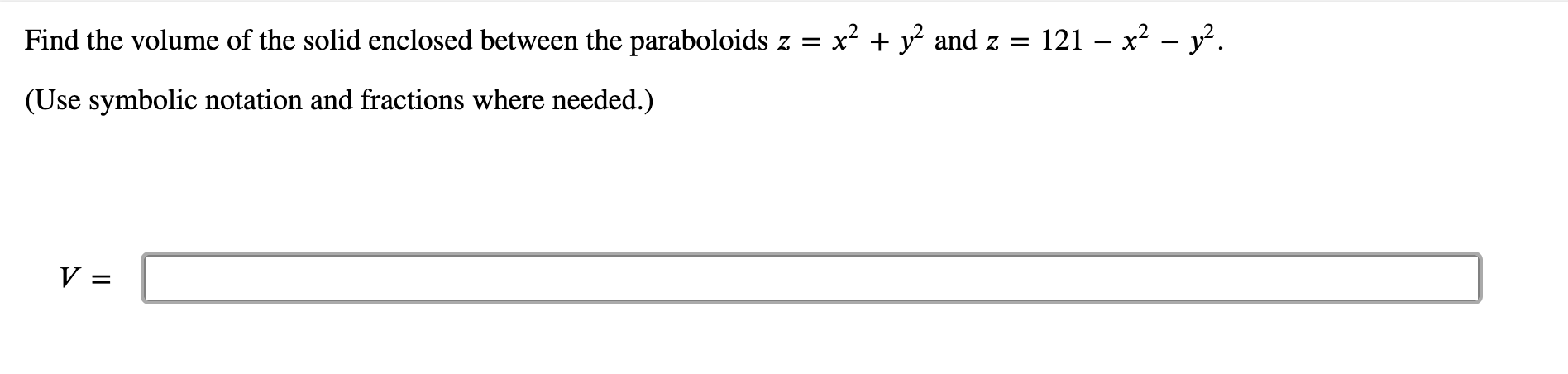Home / Expert Answers / Calculus / find-the-volume-of-the-solid-enclosed-between-the-paraboloids-z-x-2-y-2-and-z-121-x-pa816

# (Solved): Find the volume of the solid enclosed between the paraboloids \( z=x^{2}+y^{2} \) and \( z=121-x^{ ...Find the volume of the solid enclosed between the paraboloids \( z=x^{2}+y^{2} \) and \( z=121-x^{2}-y^{2} \). (Use symbolic notation and fractions where needed.) \[ V: \]

We have an Answer from Expert